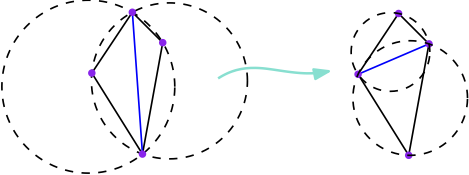# Bachelor internship: Heuristics for Computing Delaunay Triangulations on Flat Tori

Supervision and Contact: Vincent Despré and Monique Teillaud
Location: Gamble group, INRIA Nancy - Grand Est, LORIA

### Context

In the Euclidean plane, the Delaunay triangulation of a set of n points P is the unique (when P is non-degenerate) triangulation with vertex set P for which the circumdisk of each triangle does not contain any point of P in its interior. It has many applications and has been extensively studied [BCKO].
When a general (non-Delaunay) triangulation is given a sequence of edge flips allows to compute the Delaunay triangulation.The worst-case complexity of that sequence is O(n2). Moreover, that bound is tight [HNU], so, the worst-case complexity cannot be improved through smart optimizations of the algorithm.

We are interested by the generalization of the Delaunay triangulations on 2D flat tori [CT]. A 2D flat torus is the quotient of the Euclidean plane under the action of a group generated by two non-collinear translations [MT]. Thus, each point of a set P on the flat torus corresponds to a lattice in the Euclidean plane. On flat tori, the flip algorithm has a complexity O(f(T).n2), where f(T) is an explicit measure of the quality of the input triangulation T [DST]. This measure f(T) is not proven to be tight and can hopefully be improved by using a good heuristic for the order of the flips.

### Goal of the internship

Propose heuristics to optimize the flip algorithm to compute Delaunay triangulations of flat tori.

The problem may be attacked in several ways: the student may either do a lot of experiments and benchmarking or spend more time on theoretical aspects and propose more intricate heuristics (using volume of simplexes for instance [STT]) and give an actual complexity proof.

### Required skills

- Algorithms design and analysis
- C++
- Basics in maths (vector spaces, groups, topology)

### References

[BCKO] M. de Berg, O. Cheong, M. van Kreveld and M. Overmars. Computational Geometry: Algorithms and Applications. Springer-Verlag, 2008.
[CT] M. Caroli and M. Teillaud. Delaunay Triangulations of Point Sets in Closed Euclidean d-orbifolds. Discrete & Computational Geometry, 55(4):827-853, 2016.
[DST] Vincent Despré, Jean-Marc Schlenker, and Monique Teillaud. Flipping Geometric Triangulations on Surfaces. 36th Symposium on Computational Geometry, 2020.
[HNU] F. Hurtado, M. Noy, and J. Urrutia. Flipping edges in triangulations. Discrete & Computational Geometry, 22(3):333-346, 1999.
[MT] B. Mohar and C. Thomassen. Graphs on Surfaces. Johns Hopkins University Press, 2001.
[STT] D. Sleator, R. Tarjan and W. Thurston. Rotation Distance, Triangulations, and Hyperbolic Geometry. Journal of the AMS, 1986.